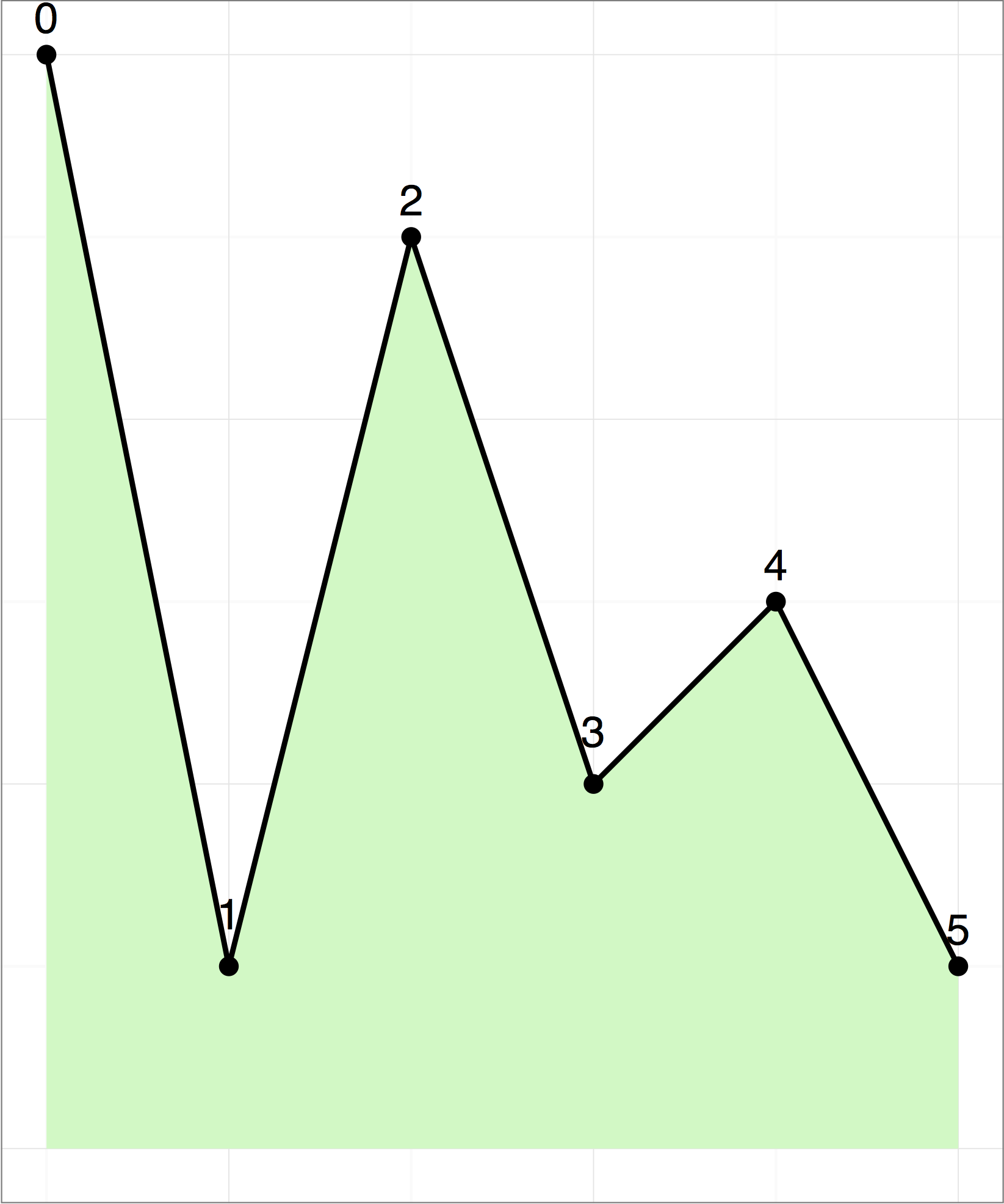시간 제한메모리 제한제출정답맞힌 사람정답 비율
1 초 512 MB52141440.000%

## 문제

Tahmuras, the third king of ancient Persia, has conquered a huge army of deevs (demons). He wants to imprison as many of them as possible in Alborz mountains and let the others go. Alborz is a mountain range with a skyline that looks like a polygonal chain with $n$ vertices. The $i$-th vertex (for all $0 \le i \le n - 1$) has coordinates $(i, y[i])$, i.e. with longitude $i$ and altitude $y[i]$.

The deevs can be imprisoned on different vertices. No two imprisoned deevs should be able to see each other; otherwise, they will make eye contact and plan to escape. Two deevs cannot see each other if there is at least one vertex between them that is strictly higher than a line connecting their vertices.

In the following figure, a deev on vertex $0$ can see deevs on vertices $1$ and $2$. But it cannot see deevs on vertices $3$, $4$ and $5$, since vertex $2$ is higher than the line connecting vertex $0$ to any of vertices $3$, $4$, or $5$.Your task is to help Tahmuras find the maximum number of deevs that can be imprisoned in Alborz mountains.

## 구현

You should implement the following procedure. It will be called by the grader once for each test case.

int maximum_deevs(int[] y)

• $y$: integer array of length $n$, the altitude of the vertices
• This procedure should return the maximum number of deevs that can be imprisoned.

## 제한

• $1 \leq n \leq 2000$,
• $0 \leq y[i] \leq 10^9$ (for all $0 \leq i < n$).

## 예제 1

Consider the polygonal chain given in the above figure.

maximum_deevs([6, 1, 5, 2, 3, 1])


The answer is $3$. One possible solution is to imprison deevs on vertices $1$, $3$ and $5$.

## 예제 2

maximum_deevs([0, 1, 2])


The answer is $1$. The deevs imprisoned on any pair of vertices can see each other (vertex $1$ is on the line connecting vertices $0$ and $2$, not strictly higher).

## 서브태스크

번호 배점 제한
1 20

$n \leq 19$

2 20

$n \leq 40$

3 30

$n \leq 300$

4 30

## 샘플 그레이더

• line $1$: $\;\;n$
• line $2$: $\;\;y \;\; y \;\; y \;\ldots\; y[n-1]$

The sample grader prints a single line containing the return value of maximum_deevs.

## 제출할 수 있는 언어

C++17, C++14, C++20, C++14 (Clang), C++17 (Clang), C++20 (Clang)

## 채점 및 기타 정보

• 예제는 채점하지 않는다.